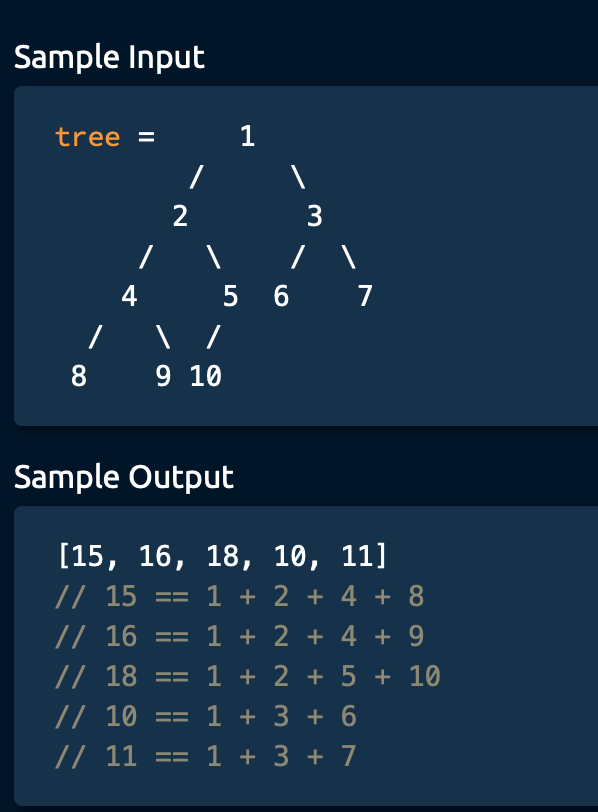# Tree Algorithm：Branch Sum1435-郭同学

, , ,

# Branch Sum

Write a function that takes in a Binary Tree and returns a list of its branch sums ordered from leftmost branch sum to rightmost branch sum.
A branch sum is the sum of all values in a Binary Tree branch. A Binary Tree branch is a path of nodes in a tree that starts at the root node and ends at any leaf node.
Each BinaryTree node has an integer value , a left child node, and a right child node. Children nodes can either be BinaryTree nodes themselves or None / null .Solution :
O(N) time | O(N) space
We are returning a list of branch sums that has the length as the number of branches.
There are roughly half of N leaf in the Binary tree, which is 1/2 N.

class BinaryTree:
def __init__(self, value):
self.value = value
self.left = None
self.right = None

def branchSums(root):
sums = []
calculateBranchsum(root, 0, sums)
return sums

def calculateBranchsum(node, runningsum, sums):
if node is None:
return
newrunningsum = runningsum + node.value
if node.left is None and node.right is None:
sums.append(newrunningsum)
return

calculateBranchsum(node.left, newrunningsum, sums)
calculateBranchsum(node.right, newrunningsum, sums)


from AlgoExpert

### 评论 抢沙发### 觉得文章有用就打赏一下文章作者

#### 支付宝扫一扫打赏#### 微信扫一扫打赏Vieu3.3主题Q Q 登 录